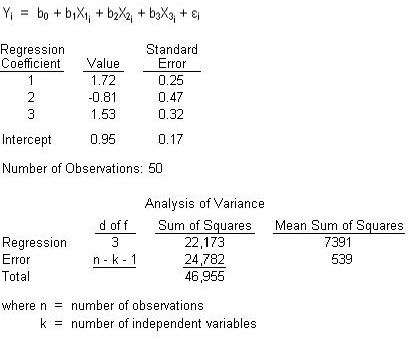### CFA Practice Question

There are 208 practice questions for this study session.

### CFA Practice Question

An analyst is currently reviewing the following regression analysis:At a significance level of 5%, which of the following statements would best reflect the null hypothesis that coefficient of the first independent variable is equal to 1.0?

A. Since the calculated t-statistic exceeds the critical t-statistic of 1.68, the null hypothesis is rejected.
B. Since the calculated t-statistic of 2.88 exceeds the critical t-statistic, the null hypothesis is rejected.
C. Since the calculated t-statistic of 6.88 falls within the critical t-statistic, the null hypothesis is accepted.

1. Set up the two-tailed t-test. H0: b1 = 1 versus H1: b1 <> 1.

2. Compute the calculated t-statistic: tcalc = (1.72 - 1.0)/0.25 = 2.88.

3. Compute the critical t-statistic (d of f = 50 - 3 - 1) t0.25, 46 = 2.013.

4. Decision: since the calculated t-statistic is greater than the critical t-statistic, the null is rejected. Therefore, the coefficient of the first independent variable is not equal to 1.0.